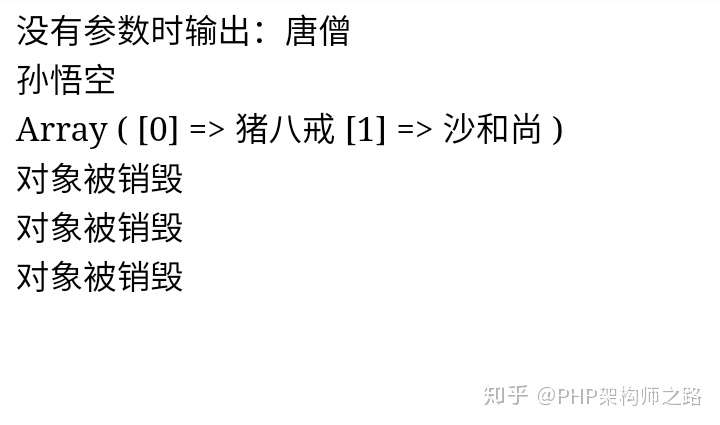# php中類的不定引數使用示例

``````<?php
/**
* __construct() 不需設定引數，用php內建函式自動獲取
*
* 在下面示例中，在建構函式裡使用了內建函式func_get_args()獲取到所有的引數，
* func_num_args()獲取到引數的數量，然後使用判斷語句，執行其中一個使用者自定義方法，
* 從而實現了建構函式帶不定引數個數的執行方法。
*/
class Person{  // 定義Person類
private \$name;  // 定義name屬性
private \$name2;

public function __construct() {
\$array=func_get_args();  //獲取所有引數
\$num=func_num_args();   //獲取引數的數量

if(method_exists(\$this, \$f='func'.\$num)){  //重要：檢查類中方法是否存在
call_user_func_array(array(\$this, \$f), \$array);  // 執行一個方法與引數陣列
}

}

public function func0() {
\$this->name = "沒有引數時輸出：唐僧";
}

public function func1(\$value) {
\$this->name = \$value;
}

public function func2(\$value1, \$value2) {
\$this->name = \$value1;
\$this->name2 = \$value2;
}

function getName(){
return \$this->name;
}

function getName2(){
\$arr = \$this->name;
\$arr = \$this->name2;
return \$arr;
}

function setName(\$value){
\$this->name = \$value;
}

function __destruct() {
print "<br>物件被銷燬";
}
}

\$person = new Person();
echo \$person->getName() . "<br>";

\$person2 = new Person("孫悟空");
echo \$person2->getName() . "<br>";

\$person3 = new Person("豬八戒", "沙和尚");
print_r(\$person3->getName2());
?>``````2021金三銀四大廠面試真題集錦，必看！

「其他文章」